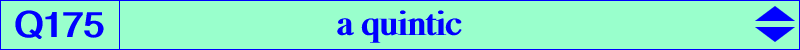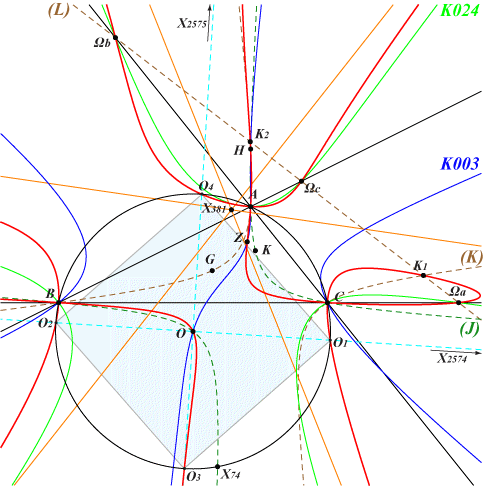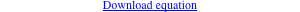too complicated to be written here. Click on the link to download a text file.X(3), X(4), X(2574), X(2575) infinite points of K024 Ωa, Ωb, Ωc : centers of the Apollonius circles K1, K2 : on the Lemoine axis (L) and the Kiepert hyperbola (K) O1, O2, O3, O4 on the circumcircle Z on K003 further details belowQ175 is a quintic having five real asymptotes : • two are parallel at O to the asymptotes of the Jerabek hyperbola (J). They meet Q175 again at four points O1, O2, O3, O4 on the circumcircle (O) which are obviously the vertices of a square. • three are parallel at X(381) to the asymptotes of the cubic K024 and to the sidelines of the CircumNormal triangle with vertices on (O) and K003. A, B, C are nodes on Q175 with nodal tangents parallel to the asymptotes of (J). The remaining points on the sidelines of ABC are Ωa, Ωb, Ωc with tangents passing through O. More generally, the locus of points whose polar line in Q175 passes through O is the quartic Q176. Q175 passes through H (with tangent passing through K) and through O (with tangent passing through X4846). *** Q175 passes through a point Z introduced in Euclid #4073 by César Lozada as follows. Let P be a point and denote byOa, Ob, Oc the circumcenters of PBC, PCA, PAB respectively. The perpendicular to POa at P intersects AB, AC at Ac, Ab respectively. Similarly define Bc, Ba and Ca, Cb. These six points lie on a conic for every P. This conic is a proper circle for one and only one point Z that lies on K003, Q018, Q098, Q157 and Q175. Indeed, P must lie on three quintics QA, QB, QC of a same pencil. Each quintic (Q) meets the line at infinity as K024 and two other points on a rectangular circum-hyperbola (H). Q175 is the quintic obtained when (H) is the Jerabek hyperbola. Note that (Q) and K024 ∪ (H) meet at 25 points on (L∞), on the sidelines of ABC and on a line passing through O which meets (H) on K003. See the analogous Q177 also passing through Z.